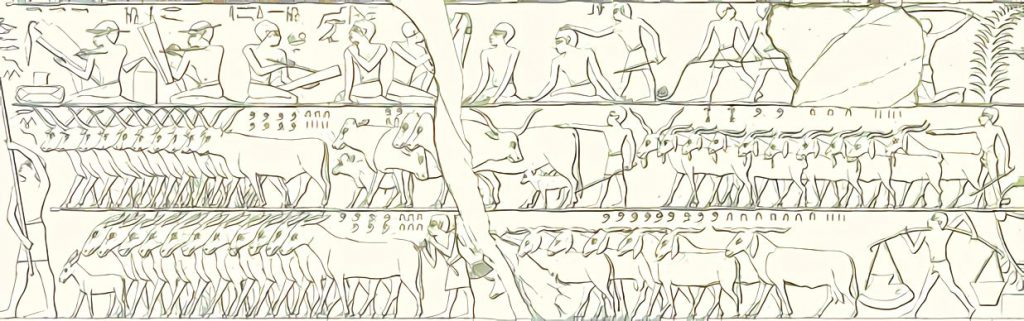# Hang in There! Why Even Algebraic Structures are Useful

Hardly any other subject can hit a freshman as hard as those strange “algebraic structures” during your first math course. You started out to solve real problems and now this professor explains to you, with a straight face, that there really exists such a thing as addition and multiplication and that surprisingly 0 is not equal to 1. What’s that got to do with the world?

For thousands of years it has proven a worthwhile activity to count things (e. g. goats) and to record the result as a number. These numbers are often added to determine the total number of all things without recounting. Even the ancient Egyptians were able to calculate that Figure 1 shows a total of 3209 (=2235+974) goats.Fig 1: This scene depicts a cattle count (copied by the Egyptologist Lepsius). In the middle register we see 835 horned cattle on the left, right behind them are some 220 animals (cows?) and on the right 2235 goats. In the bottom register we see 760 donkeys on the left and 974 goats on the right (enhanced version of the original on Wikipedia using waifu2x).

Sometimes the multiple of a number is of interest (e. g. “If 20 warriors fit on one ship, how many warriors fit on 7 ships?”), which is called multiplication. Addition and multiplication must sometimes be reversed (subtraction and division). So far so good. But actually, we already learned that in primary school.

## Objects For Mathematical Operations

Numbers are objects on which mathematical operations can be performed. Addition, subtraction, multiplication and division are examples of this, but there are other more complex operations and objects.

An example of this is the largest common divisor. The Euclidean algorithm, that calculates it, works on integers. Other mathematical objects such as polynomials can also be used, as long as a division with remainder (also called Euclidean division) is possible. Such mathematical objects are called Euclidean rings.

In Figure 2 some algebraic structures are shown using a class diagram, as used in JLinAlg – an open source library for linear algebra implemented in Java. There is also an implementation of the Euclidean algorithm in JLinAlg that can be applied to objects implementing the interface IEuclideanRingElement. Thus, the Euclidean algorithm works analogously to integers also for polynomials (class Polynomial), but not for objects of type Matrix and in general not for fields (i.e. subclasses of FieldElement).

## What it Really is All About

Just as most people can add up apples in the same way as oranges, a student of STEM subjects (i.e. mathematics, computer science, natural sciences and technology) should learn to combine all kinds of objects:

• Connections from timetables, roads or communication networks
• Electronic circuits
• Private and public keys when signing and encrypting data
• Controls such as text input fields and buttons
• Steps within a process for process optimization
• Objects in programming languages

Of course, most of the time we are not dealing with algebraic structures, but the basic idea is always the same. For all these operations/algorithms, there are conditions, and if the properties of the underlying structures are similar enough, procedures that can be applied to one problem can also be applied to another. The shortest route, for example, can be found using a map as well as with the help of a timetable.

With many problems it requires a little bit more effort so that a well-known procedure leads to the solution of a new problem. This can be achieved e.g. by using a mathematical transformation of the problem definition, as described in the article Problem Reduction – The Most Reliable Path is Just Another Shortest Path (see also Figure 3).Fig. 3: How to find the most reliable way? Using a little trick the solution is easier than expected.

## Appeal to all STEM students

I realize that it is difficult to motivate yourself for lectures like Linear Algebra, Analysis or Stochastics. Your brains need to be reconfigured a bit – and that can be painful. But sometimes it’s also pretty cool what you can teach your brain.

The ways of thinking you learn are abstract – sometimes quite distant from real world applications, but the more you know and keep in your mind (!), the less bumbling your solutions will be in your working life. Hang in there! It’s totally worth it.

### Related Posts

This entry was posted in JLinAlg, Mathematics, Open Source on August 20, 2020.